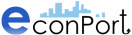http://www.econport.org   A Digital Library for Microeconomics Education Copyright © 2006 Experimental Economics Center, Georgia State University. All rights reserved.Experimental Discussion of the St. Petersburg Paradox Structure of the Game Subjects are made an offer. A fair coin will be tossed continuously until it turns up tails. If the coin comes up tails on the nth toss, the subject will receive \$2n, i.e. if it comes up tails on the 5th toss, the subject receives \$25 = \$32. Simulating the Game Using a Computerized Experiment Performing the experiment with an actual coin could get quite tedious and complicated, especially with a large number of subjects, but it is fairly easy to simulate using a computerized experiment. For example, one can simply draw from a binary, i.e. (0,1) valued uniform distribution, and assign payoffs when the draw equals 1. The specifics of the algorithm would, of course, depend on one's language of choice. Average payoffs could be calculated by storing the payoffs of each game, summing them, and dividing by the number of times the game was played. Results of Experiments Conducted Jerger (1992) refers to empirical investigations which show ?1, or one Euro, as the upper boundary that people are willing to pay in order to participate in the game. This extremely low value is probably due to diminishing marginal utility of income, and a significant degree of risk aversion, resulting from the very small probability of actually winning a very high payoff. Hinners-Tobrägel (2003) discusses the results of a computerized simulation of the game. The average payoff after 10 repetitions of the game was ?2.1. However, when the game was repeated many more times, the average payoffs started to vary dramatically, from ?4.5 after 250 repetitions, to ?5.8 after 1,000 repetitions, to ?13.7 after 2,500 repetitions. This is simply because the expected value of the game is infinite, so of course the expected value of the sample mean is also infinite. In the 1700s, Buffon actually conducted the experiment with a child, for 211 = 2048 repetitions. (How he got a child to stay in one place for such a long time is a question best left unasked.) He found that the number of plays of length 1, 2, 3, 4, 5, 6, 7, 8, and 9 was 1061, 494, 232, 137, 56, 29, 25, 8, and 6 respectively. The expected values of these numbers are 1024, 512, 256, 128, 64, 32, 16, 8, and 4 respectively. The average payoff was 4.91. References
 Page source: http://www.econport.org/econport/request?page=man_ru_experiments_stpetersburg